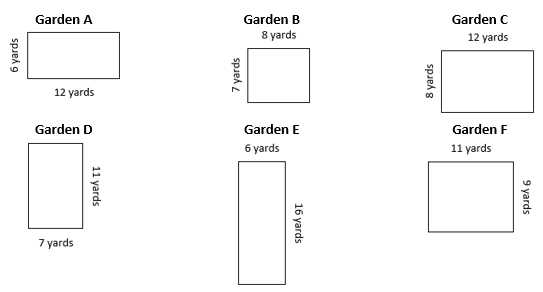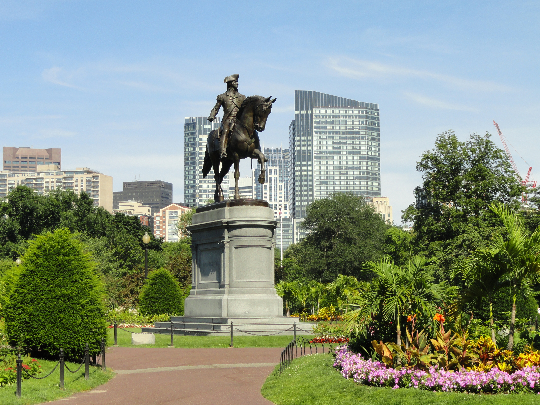# Multi-Digit Multiplication

## Objective

Abstract the formulas for the area and perimeter of a rectangle and apply those formulas in real-world and mathematical problems involving multiplication, addition, and subtraction.

## Common Core Standards

### Core Standards

?

• 4.MD.A.3 — Apply the area and perimeter formulas for rectangles in real world and mathematical problems. For example, find the width of a rectangular room given the area of the flooring and the length, by viewing the area formula as a multiplication equation with an unknown factor.

• 4.OA.A.3 — Solve multistep word problems posed with whole numbers and having whole-number answers using the four operations, including problems in which remainders must be interpreted. Represent these problems using equations with a letter standing for the unknown quantity. Assess the reasonableness of answers using mental computation and estimation strategies including rounding.

## Criteria for Success

?

1. Generate the formula for the area of a rectangle: A = l x w.
2. Generate the formula for the perimeter of a rectangle: P = l + w + l + w or P = 2l + 2w or P = 2(l + w).
3. Solve real-world and mathematical problems involving area and perimeter that require multiplication, addition, and/or subtraction to solve (MP.4).
4. Understand that when finding areas of rectangular regions answer will be in square units, and when finding the perimeter of a rectangular region, answers will be in linear units (MP.6).

## Tips for Teachers

?

• Because of the extensive work with the area model in this unit, as well as the work students did with area and perimeter in Grade 3, this lesson starts with abstract models of area rather than unitized models where individual square units can be seen (e.g., on graph paper). If you think it would be beneficial for some students in class to see individual units, you could modify Anchor Tasks #1 and #2 so that the rectangles are drawn on graph paper and then provide graph paper for students to use throughout the remainder of the lesson, if need be.
• This lesson involves students “repeatedly reasoning about how to calculate areas and perimeters of rectangles, [allowing] students [to] come to see area and perimeter formulas as summaries of all such calculations (MP.8)” (MD Progression, p. 21). Then, students will apply these formulas in the context of real-world and mathematical problems involving multiplication, area, and perimeter. Problems involving area and perimeter that rely on division (e.g., unknown side length problems) will come in Unit 3.
• “When engaging in the mathematical practice of reasoning abstractly and quantitatively (MP.2) in work with area and perimeter, students think of the situation and perhaps make a drawing. Then they recreate the “formula” with specific numbers and one unknown number as a situation equation for this particular numerical equation” (GM Progression, p. 22)
• This lesson provides an opportunity to connect two domains in the grade, 4.OA and 4.MD, since students will be solving multi-step word problems that involve area and perimeter, thus connecting standards 4.OA.3 and 4.MD.3.

#### Remote Learning Guidance

If you need to adapt or shorten this lesson for remote learning, we suggest prioritizing Anchor Task 3 (benefits from worked example). Find more guidance on adapting our math curriculum for remote learning here.

#### Fishtank Plus

• Problem Set
• Student Handout Editor
• Vocabulary Package

?

### Problem 1

The City of Boston is thinking about creating a new flower bed in Boston Public Garden. Here are the different potential plans they’ve come up with:1. What is the area of each garden that the city is considering?
2. What general formula could you write for all of the calculations you did in Part (a)?

### Problem 2

The City of Boston would like to put a chain fence around the garden, like the one around the Paul Revere statue pictured below:a. How much chain would they need for each of the garden plans above?

b. What general formula could you write for all of the calculations you did in Part (a)?

#### References

Wikimedia Commons Photo: Boston Public Garden - Boston, MA - DSC01502.JPG by Daderot

Boston Public Garden - Boston, MA - DSC01502.JPG is made available on Wikimedia Commons under the CC0 1.0 license. Accessed Dec. 17, 2018, 9:51 a.m..

### Problem 3

Mrs. Allen is redecorating her living room. Her living room is 15 feet long and 22 feet wide. She wants to replace the carpet for $7 per square foot and replace the baseboards for$3 per foot. How much will it cost to replace the carpet and baseboards?

## Problem Set & Homework

#### Discussion of Problem Set

• What units did you include for the area and perimeter of each figure in #1? Why did you use those units?
• Did the tiles correspond with the area or perimeter of Josie’s kitchen in #6? What about the wood? Why?

?

Karl’s rectangular vegetable garden is 20 feet by 45 feet, and Makenna’s is 25 feet by 40 feet.

1. Whose garden is larger in area? By how much?
2. Whose garden is larger in perimeter? By how much?

### Mastery Response

?